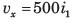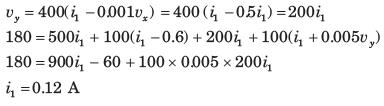Courses

# Test: Network Equation (Mesh & Node) - 2

## 7 Questions MCQ Test Network Theory (Electric Circuits) | Test: Network Equation (Mesh & Node) - 2

Description
This mock test of Test: Network Equation (Mesh & Node) - 2 for Electrical Engineering (EE) helps you for every Electrical Engineering (EE) entrance exam. This contains 7 Multiple Choice Questions for Electrical Engineering (EE) Test: Network Equation (Mesh & Node) - 2 (mcq) to study with solutions a complete question bank. The solved questions answers in this Test: Network Equation (Mesh & Node) - 2 quiz give you a good mix of easy questions and tough questions. Electrical Engineering (EE) students definitely take this Test: Network Equation (Mesh & Node) - 2 exercise for a better result in the exam. You can find other Test: Network Equation (Mesh & Node) - 2 extra questions, long questions & short questions for Electrical Engineering (EE) on EduRev as well by searching above.
QUESTION: 1

### i1 = ?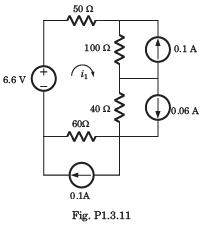Solution: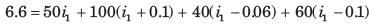i1 = 0.02 A

QUESTION: 2

### For the circuit of Fig. P1.3.38 the value of vs, that will result in v1 = 0, is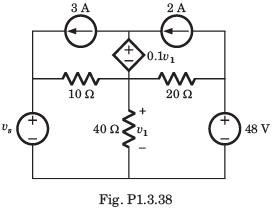Solution:

If v1 = 0, the dependent source is a short circuit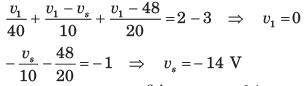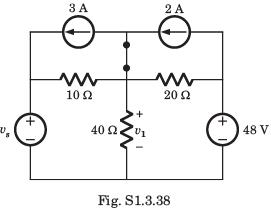QUESTION: 3

### i1, i2 = ?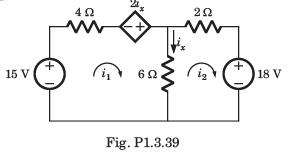Solution:

ix = i1 - i2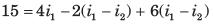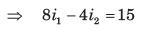....(i)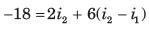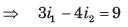...(ii)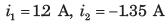QUESTION: 4

v1 = ?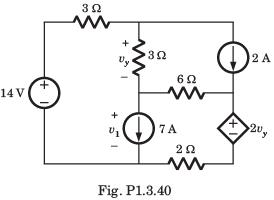Solution: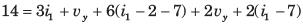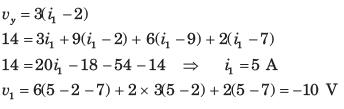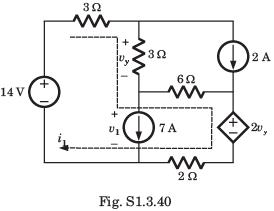QUESTION: 5

vx = ?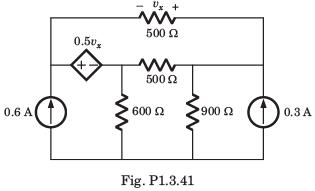Solution:

Let i1 and i2 be two loop current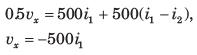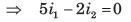...(i)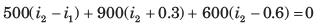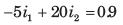...(ii)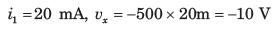QUESTION: 6

The power being dissipated in the 2Ω resistor in the circuit of Fig. P1.3.42 is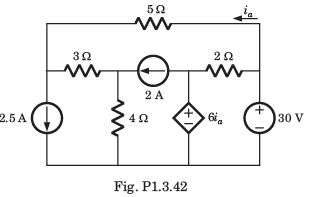Solution: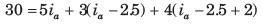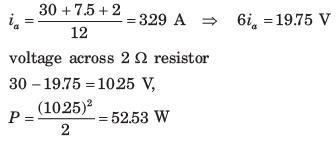QUESTION: 7

i1 = ?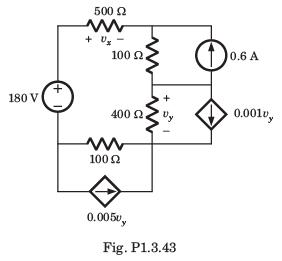Solution: